Win up to 100% scholarship on Aakash BYJU'S JEE/NEET courses with ABNAT Win up to 100% scholarship on Aakash BYJU'S JEE/NEET courses with ABNAT

# JEE Maths Study Material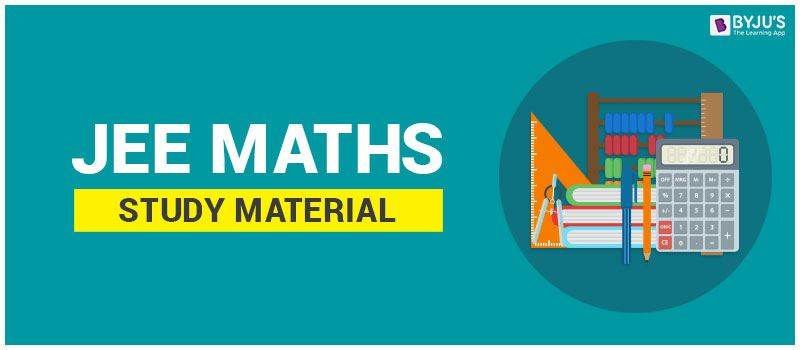IIT JEE Maths Study Material – To be an expert in JEE Mathematics, it is absolutely necessary to practice and be familiar will all the concepts as well as the questions of different types. This is essential to gain mastery over the subject. We have also often heard the common saying, “Practice Makes a Man Perfect”, hence students have to practice, practice and practice till they master the subject.

Once they get enough practice, students will naturally be excited to attempt all questions in one shot. This approach, however, would work better if students are pretty sure about the answers in the question paper. A better strategy would be to categorize questions as per their difficulty level viz. easy, medium and difficult ones. Begin with the easy question and then to the medium ones and save enough time to attempt the difficult questions. The best part of this strategy is that it would help students to score higher while avoiding the possibility of negative marks.

Download Free PDFs for Daily Practice Problems and Worksheet for Mathematical Tools

Students can boost their IIT JEE Maths preparations by referring to the study materials provided in the table below:

## JEE Maths Study Material

Students can click on the links given on the table and access detailed information about the different topics.

### JEE Calculus

Apart from strategizing the answer plan, time management has a direct influence on the JEE score. To score well in the exam, it would be ideal to solve the majority of math questions within 1 hour and 20 minutes.

## Weightage Of Topics

Let us look at some of the important topics covered in the recent exam.

 Maths Topics Weightage of Marks Sets, Relation and Function 4 Sequences and Series 4 Binomial Theorem and Its Application 4 Differential Equation 4 Differential Calculus 4 Permutations and Combinations 4 Trigonometry 4 Mathematical Reasoning 4 Statistics and Probability 8 Matrices and Determinants 8 Vector Algebra 8 Three Dimensional Geometry 8 Complex numbers and Quadratic Equation 8 Limits, Continuity and Differentiability 12 Integral Calculus 12 Coordinate Geometry 20 Total 120

Mathematics is one of the wonderful creations of the human mind that helps us to build our reasoning capabilities. IIT JEE syllabus for maths contains important topics like algebra, trigonometry, calculus and so on which should be attentively covered by students. Here are some important topics students have to watch out for:

Algebra:

The topic of Algebra is known to all, owing to the same being one of the core foundational topics in the subject. Also recognized as one of the high scoring domains for JEE Maths, this topic is a really interesting one and requires good computational speed. To excel in this domain, students have to understand the basic concepts clearly to tackle tricky questions. The important topics that come under algebra include:

Calculus:

In JEE maths, Calculus is undoubtedly the most important topic for the JEE exam as this topic has the largest influence on modern-day mathematics. There are two branches for this domain viz. integral and differential calculus. The study of calculus could be termed as the study of change which is quite similar to geometry which is the study of shapes and study of operations done by algebra. The main topics under calculus include:

Trigonometry:

The domain of Trigonometry is recognized by everyone as one of the core math topics and has its own significance in the JEE Maths section. The ratios and functions of trigonometry are used frequently in questions during the exam. Once students have a good grip on the basic concepts in this topic, they will be on the way to score really well in the exam. For further details on JEE mathematics, tips and videos lectures on how to crack the exam, visit BYJU’S.

### 100 Most Important Maths JEE Main Questions – Part 1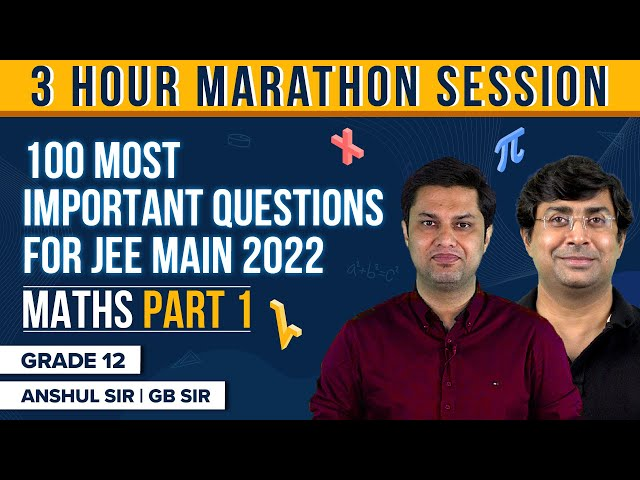### 100 Most Important Maths JEE Main Questions – Part 2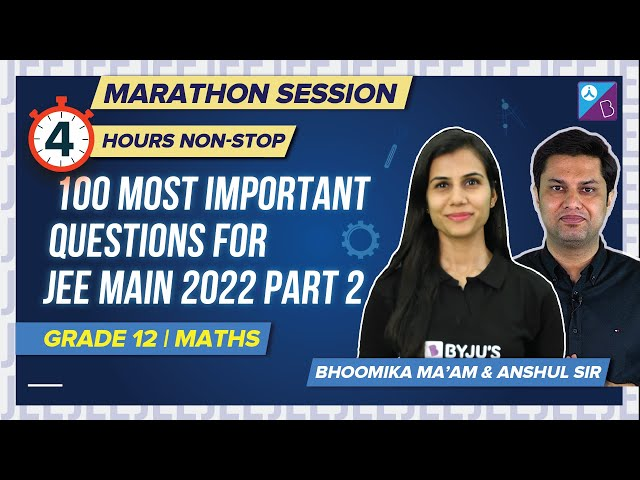## 5 Common Mistakes to Avoid in JEE Maths Exam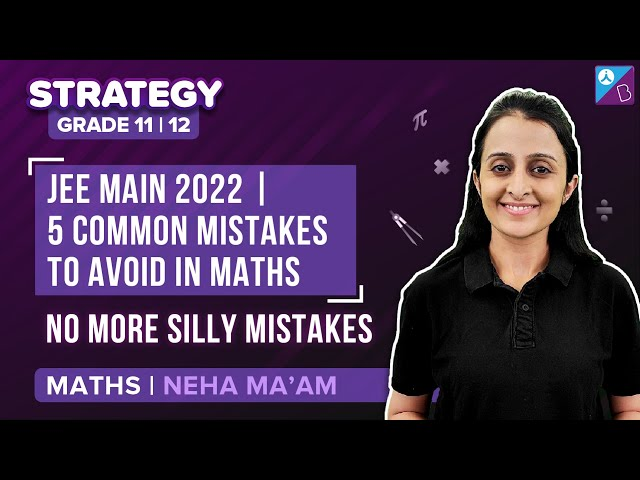## JEE Maths Preparation Tips

Maths is an important section in JEE. Apart from being a scoring subject whenever there is a tie in the exam then the score obtained in this section is used to decide who gets a better rank. Therefore, candidates should focus on performing well in this section. However, they can perform excellently only if they have prepared expertly for the exam. They should be well versed with the topics, have a clear understanding of the concepts and they should possess adept problem-solving as well as time management skills. Once they develop these things they will be paving the way to success. To help candidates give their best and achieve an outstanding result we have put together some of the most effective preparation tips for JEE. Aspirants can follow these tips and study more productive for the exams.

• Focus On The Important Topics

In JEE Maths there are usually seven topics that are important and candidates should study these topics thoroughly. The topics are 3-D geometry, probability and statistics, vector algebra, integration, complex numbers, parabola, and trigonometric ratios. Learning these topics will enable students to score higher marks in the exam.

• Choose Only The Best Resources

It is crucial for candidates to choose only those resources which will add to their JEE preparation. They should make use of the right and best maths books for JEE and study from them. Some of the recommended books are NCERT, S.K. Goyal, S.L. Loney, R.D. Sharma, amongst others. These books usually contain questions and exercises based on the JEE syllabus and format.

• Solve Question Papers And Practice Often

Try to solve as many question papers as possible. It can be past papers, model papers or just sample papers. Get hold of them and start practising. The solutions are usually provided with the questions but we recommend candidates to first solve the question paper and then check the answers. Additionally, they can attempt the mock tests and they should keep a close track of the time.  All these will help candidates to test their preparation level and also know the question paper format, types of questions, marking scheme, exam duration, etc. Practising more is key to getting better at solving problems and developing enhanced maths fluency.

• Focus On The Accuracy Of Answers

While studying maths candidates need to realize that accuracy is better than speed. While it is important to solve the questions in time but if it is not accurate then it is a loss. Therefore, candidates should focus on answering the question more accurately as they can help them score higher marks. Besides, if candidates attempt 100% of the questions but they are incorrect, then they will lose marks. Maths questions are quite tricky and complicated and guesswork will definitely not work. Understand the concepts and their application in different problems.

• Stay Motivated

JEE preparation is a long process. It usually begins at least a year in advance before the final exam. In this, aspirants need to stay motivated to learn and put in the same effort throughout the preparation stage. They should have a positive mindset and not take any stress.

## How to Study Maths for JEE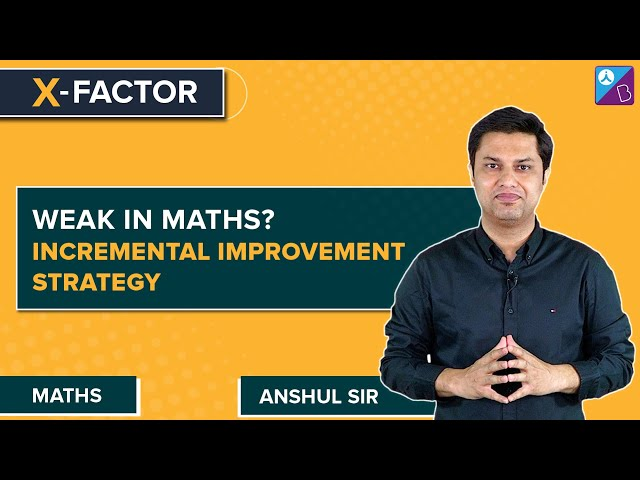## Maths Sure Shot Topics for JEE Main 2022/23### Guaranteed and Important 12 Marks JEE Mains Maths Questions### Most Difficult IIT JEE Maths Questions### How to Solve Conceptually Challenging JEE Maths Problems?## Frequently Asked Questions On IIT JEE Maths Study Material

Q1

### Which are the best books for JEE maths?

Some of the most useful books for learning IIT JEE maths are books from authors such as R.D. Sharma, S.K. Goyal, Arihant publications, Amit M. Agarwal, amongst others.

Q2

### Is R.D. Sharma enough for JEE Mains?

Well, having more than one useful study material is always better than studying from one resource. As such, R.D. Sharma is a great book to start your JEE preparation for maths. This will help you understand the concepts in-depth as well as develop better math fluency. The books contain all levels of questions based on JEE exams.

Q3

### In what sequence should I study maths for JEE?

While the study method solely depends on the individual candidate, one of the effective ways which students can follow is to study topic-wise. They can follow the sequence; Start with calculus, then move onto trigonometry and algebra and finally get into learning the conics part. Remember to complete the syllabus in time and also have enough time for revisions.

Q4

### Is NCERT a good book to study maths for JEE Main?

NCERT is a good book to study from for JEE. It will help you develop a better understanding of the topics as well as math fluency. However, you should also refer to some additional math textbooks as these will further cover the topics in more detail and the problems will be based mainly on JEE format. You can, therefore, learn more productively and sharpen your problem-solving skills.

Q5

### How to study maths for JEE?

The best way to study maths and master concepts is to practice solving sums a lot. However, it is equally important to understand the concept clearly and then apply the knowledge to solve the problems. With maths, candidates should understand the questions like how and why when solving the numerical. Use question papers as well as attempt mock tests to get a better idea of the math questions and the pattern of JEE exam.